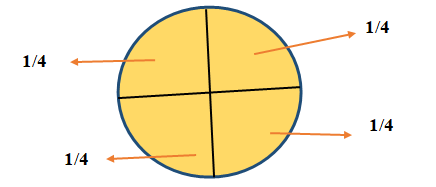# Unit Fraction

In mathematics, when we divide a complete part into some equal parts then each part shows the fraction of whole part. Like pizza, pizza is a circular in shape, if we divide it in 8 equal parts then each part is the fraction of whole part.

Fractions are written in the form of ratio of two numbers. The upper number is called as numerator and the number below is called as denominator.

For example: a/b is the fraction, in which a is numerator and b is the denominator.## Unit fractions:

Unit fractions are the fractions in which numerator is always one and denominator is any whole number except zero.

For example:

a/b is the unit fraction only if a= 1 and b≠0.

1/5, 1/8, 1/12 all are the unit fractions, because here numerator is one.Here, numerator is 1 and denominator is 8≠0.

If a complete circle is divided into 4 equal parts then each part will be the fraction of whole part. And each part takes the value as ¼ and it is the unit fraction.• Here, complete part / total equal parts = 1/4
• Here, each part contributes ¼ th part of the whole part and it is in the unit fraction form.
• And total part of circle = ¼ + ¼ + ¼ + ¼ = 1

• Similarly, if we divide a complete circle into 8 equal parts then each part will contribute the 1/8th part of the complete part and it is in the form of proper fraction as numerator is less than denominator.
• Here, each fraction takes value 1/8 because, complete part/ number of equal parts = 1/8
• And hence, 1/8 + 1/8 + 1/8 + 1/8 + 1/8 + 1/8 + 1/8 + 1/8 = 1The following figures shows the different fraction on the basis of the portion selected out of complete part.Here, in figure there is a complete circle hence it takes Value 1• In fig. 2 we divided the complete circle into 2 equal parts hence each part takes the value ½.

Since, complete part/ number of equal parts = ½, and it is the proper fraction.• In fig. 3 the whole circle is divided into 3 equal parts and hence each part takes value 1/3 and it is the proper fraction.• In fig. 4 the whole circle is divided into 4 equal parts hence each part takes the value ¼ and it is the proper fraction.• In fig. 5, the whole circle is divided into 5 equal parts and each part takes the value 1/5, and it is the proper fraction.• Also in fig. 6, the whole circle is divided into 6 equal parts hence, each part takes the value 1/6 and it is the proper fraction.

Note:

As the numerator is less than denominator in proper fraction, the decimal value of proper fraction is always less than 1.

Updated: July 15, 2021 — 12:06 am